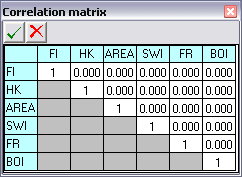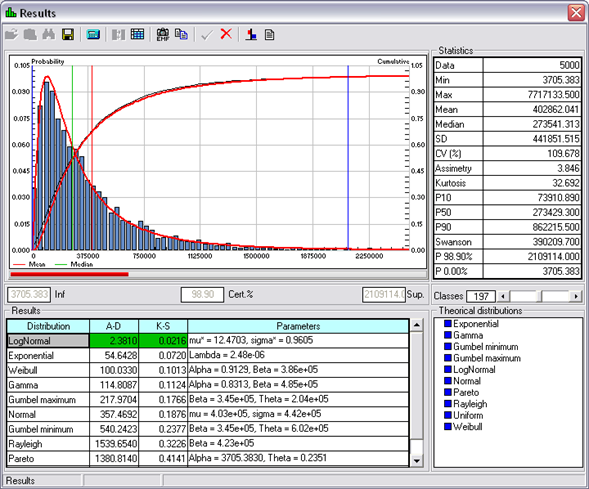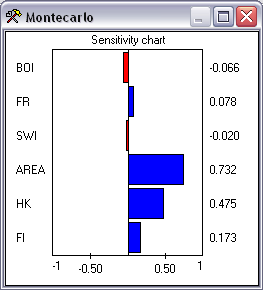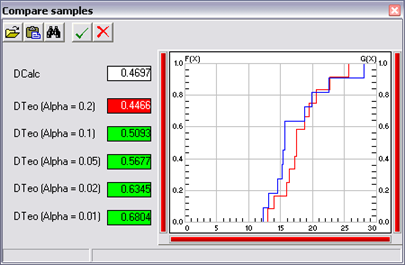### Montecarlo Simulations and Statistical Analysis

Make any stochastic calculation in Sahara´s Montecarlo Simulator using traditional Montecarlo or Latin Hypercube calculations.• Choose from variety of 17 statistical distributions as input hypothesis (continuous or discrete).
• Assign input distributions determining their parameters, percentiles or fitting the best distribution from collected data.
• Truncate distributions if necessary.
• Check your input with the preview.
• Study how parameters affect each distributionCheck how correlation between input variables can influence your simulation results.Montecarlo Simulation Results are shown in Sahara´s Statistical Analysis tool where you can:

• Display your results in a histogram to estimate probability density function.
• Analyze data from statistical measures
• Edit data.
• Change the number of histogram classes
• See how much probability is bounded between movable percentile bars.
• Fit the best theoretical distribution to data.
• Draw estimated theoretical distributions over sample graphics.Montecarlo Statistical Analysis tool is also available in Sahara from mapped data (from polygons tool) and as individual tool. Soon available from Log Data!!

###### Brochures

STATISTICAL TOOLS